## Maxwell Bridge Theory:

Maxwell Bridge theory, shown in Fig. 11.21, measures an unknown inductance in terms of a known capacitor. The use of standard arm offers the advantage of compactness and easy shielding. The capacitor is almost a loss-less component. One arm has a resistance R1 in parallel with C1, and hence it is easier to write the balance equation using the admittance of arm 1 instead of the impedance.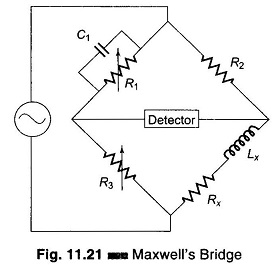The general equation for bridge balance is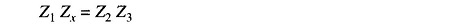i.e.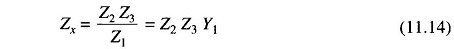where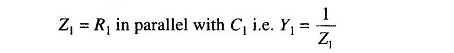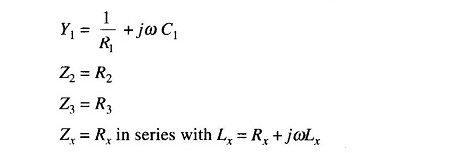From Eq. (11.14) we have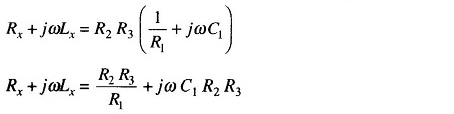Equating real terms and imaginary terms we have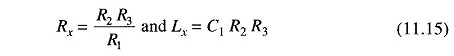Also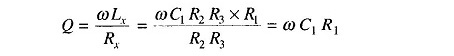Maxwell Bridge Theory is limited to the measurement of low Q values (1 – 10). The measurement is independent of the excitation frequency. The scale of the resistance can be calibrated to read inductance directly.